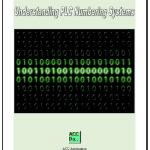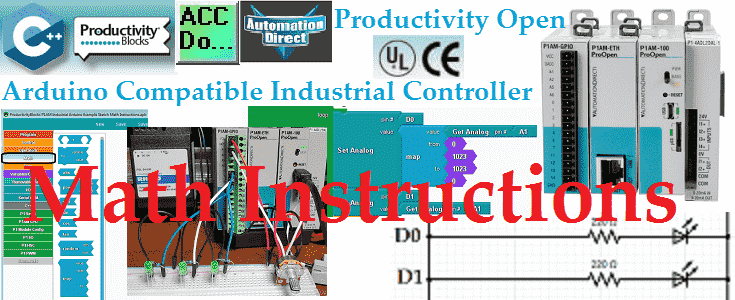# Productivity Open P1AM Industrial Arduino Math

Math instructions in your Arduino sketches (programs) consist of arithmetic operations, compound operators, absolute, power, square root, sin, cos, tan, random, map and constrain.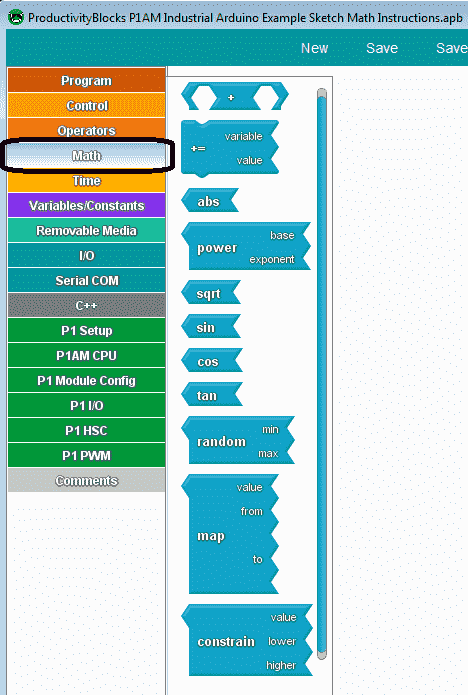These instructions will be generally used with other instructions in your sketch.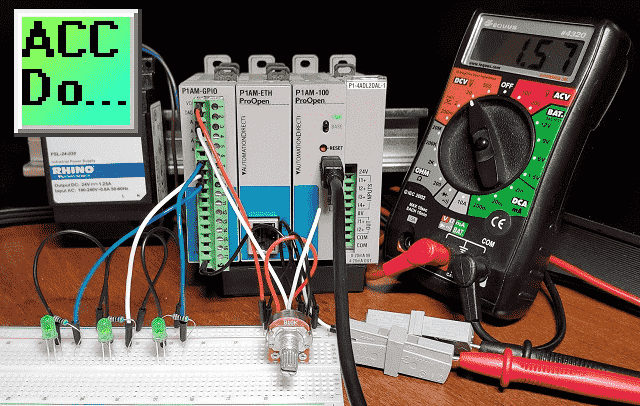We will be looking at each of these instructions that are available using productivity blocks. A sample program will be discussed that will involve some of these math instructions. Let’s get started.

Previous posts in this Productivity Open Arduino Compatible Industrial Controller Series
A full list can be obtained at the following location:
Productivity Open (P1AM-100) Arduino Controller
Productivity Open Arduino Controller Hardware
– Starter Kit Unboxing Video
Powering Up Video
Installing the SoftwareVideo
First ProgramVideo
Program StructureVideo
Variables Data TypesVideo
Serial Monitor COMVideo
Program ControlVideo
OperatorsVideo
GPIO Inputs and OutputsVideo

Watch the video below to learn about the math instructions of our productivity open industrial arduino controller.

##### Arduino Arithmetic Math Operations

Performs basic mathematical operations on numeric variables and/or constants.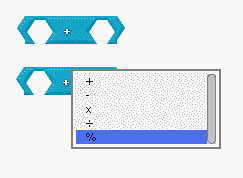You place two numeric values with the arithmetic operator and then use the dropdown selection to choose the operation.
– Subtract the values
x Multiply the values
/ Divide the values
% Remainder – This is the remaining value after the values are divided.
Example: 2 = 7 % 5

##### Arduino Compound Math Operators

Set a variable equal to the result of an operation involving itself and another number. Place a numeric variable inside the ‘variable’ socket, and another numeric value in the ‘value’ socket. Use the dropdown to select the desired operation.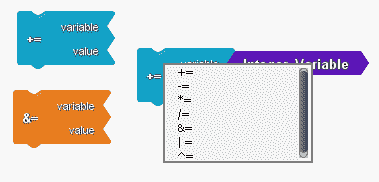The following are the dropdown selection in the order they appear.
&= Compound Bitwise AND
-= Compound Subtraction
*= Compound Multiplication
/= Compound Divide
|= Compound Bitwise OR
^= Compound Bitwise XOR (one or the other but not both)
0011 binary = 3 decimal – operand 1
0101 binary = 5 decimal – operand 2
0110 binary = 6 decimal = operand 1 XOR operand 2

##### Arduino Abs – Absolute Math

Return the absolute value of the input.The absolute value of the input is equal to the distance from 0. It will always return a positive number.
If x = 5 then abs(x) equals 5.
If x = -5 than abs(x) equals -(-5) = 5

##### Arduino Power Math

Raise a number to a power.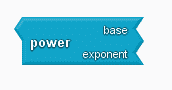Calculates the value of a number raised to a power. pow() can be used to raise a number to a fractional power. This is useful for generating an exponential mapping of values or curves.

##### Arduino Square Root – sqrt Math

Return the square root of the input.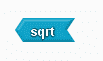If x =16 then sqrt(x) equals 4.

##### Arduino Sine, Cosine, Tangent (sin, cos, tan) Math

Sine, Cosine, and Tangent are the main functions used in Trigonometry and are based on a Right-Angled Triangle.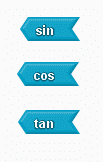Calculates the sine, cosine, or tangent of an angle (in radians). The result will be between -1 and 1.

##### Arduino Random Number

Return a random number from a range of integer values.The block will return a random number between ‘min’ and ‘max’-1.

##### Arduino Map (Scaling) Math

Scale the ‘input’ from a given range into a given output range.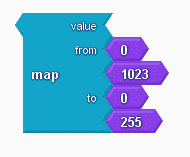The ‘value’ is the value to be scaled. The numbers in the ‘from’ sockets determine the range of the input ‘value’ and the numbers in the ‘to’ sockets determine the range of the output. A result is a number in the ‘to’ range that corresponds to the ‘value’ in the ‘from’ range.

##### Arduino Constrain Number

Restrict an output to have a value in a particular range.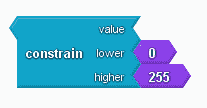The block will return a value between ‘lower’ and ‘higher’ based on the input ‘value’.

##### Sample Arduino P1AM program using map (scaling) and random math

Our sample program will take the analog input A1 and output this to D0 and D1 using PWM. (pulse width modulation) Using the map function we will do the exact opposite for D0 compared to D1.
The sample program will also print a random number between 0 and 1024. (0 to 1023) This will then control the PWM output to D2.

Wiring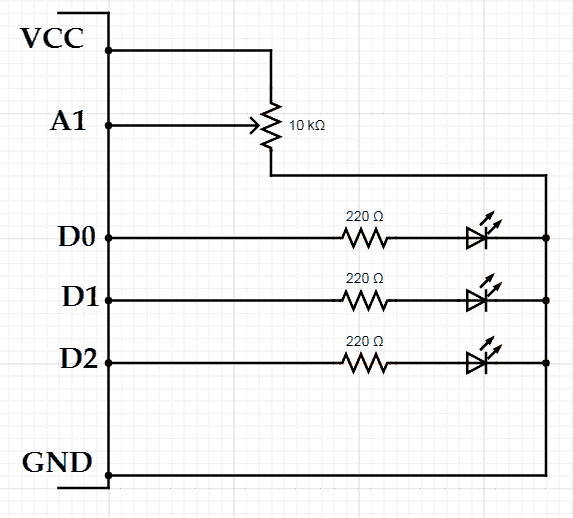Note: This is going to the GPIO industrial shield for the P1AM. Most inputs are protected, but we have included 220-ohm resistors for the LED outputs.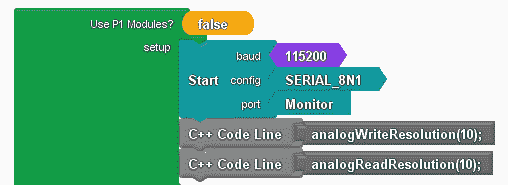Initialize the serial monitor and set the analog input and output to 10-bit resolution. (0 to 1023)

```int __ardublockAnalogRead(int pinNumber)
{
pinMode(pinNumber, INPUT);
}
{
pinMode(pinNumber, INPUT);
}
int _PBVAR_1_Integer_Variable = 0 ;

void setup()
{
Serial.begin(115200, SERIAL_8N1);
analogWriteResolution(10);

}```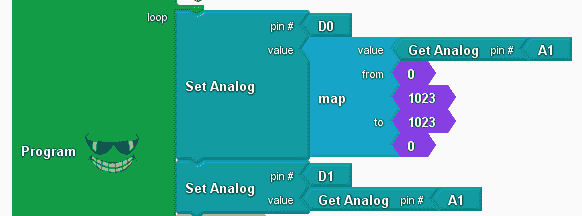Use the map math instruction to scale the analog input (A1) for D0. As the input goes from 0 to 1023 the PWM on D0 will go from 1023 to 0. This will be the opposite of the PWM output for D1.

```void loop()
{
analogWrite(0 , map ( __ardublockAnalogRead(A1) , 0 , 1023 , 1023 , 0 ) );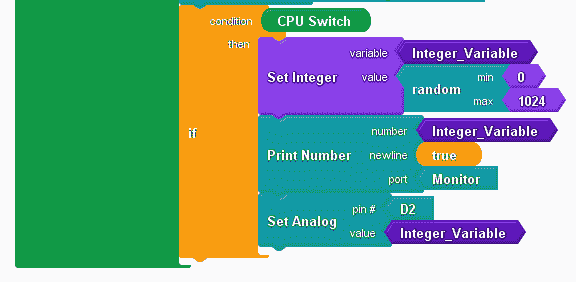If the CPU switch is on, then generate a random number between 0 and 1024. This will give us the value to print on the monitor and set the PWM output on D2.

```if (__proBlocksDigitalRead(31))
{
_PBVAR_1_Integer_Variable = random( 0 , 1024 ) ;
Serial.println(_PBVAR_1_Integer_Variable);
analogWrite(2 , _PBVAR_1_Integer_Variable);
}
}```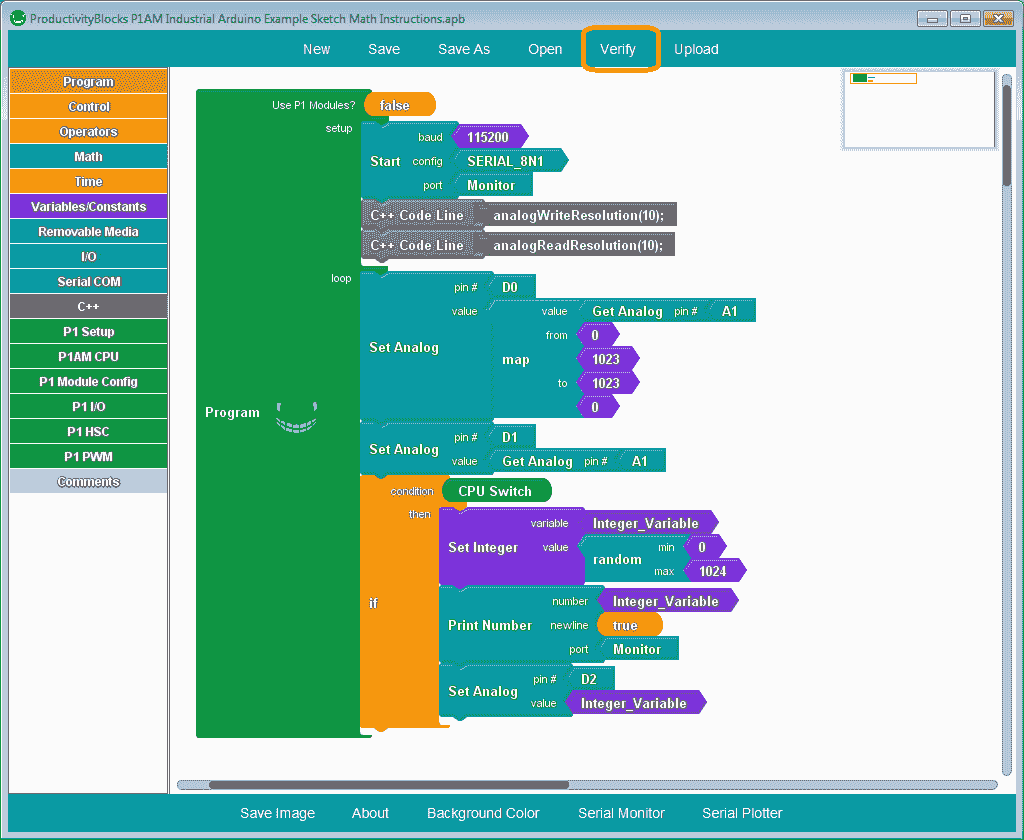Here is the complete program. Click the verify button to compile and translate our program to the C++ code for our P1AM-100 CPU.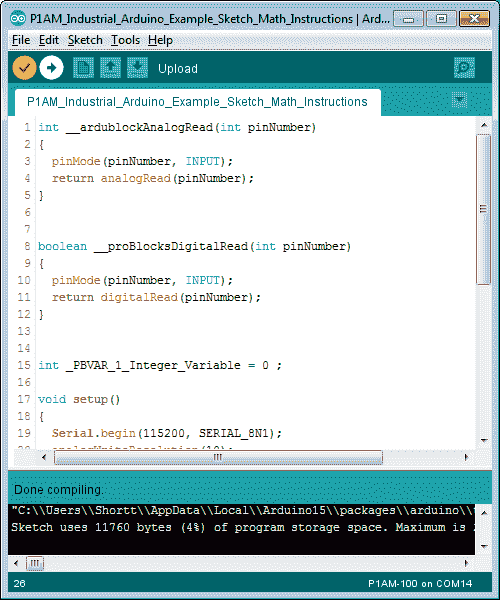Once the arduino software (IDE – integrated development environment) is finished compiling, select the upload button to send the program to our P1AM CPU.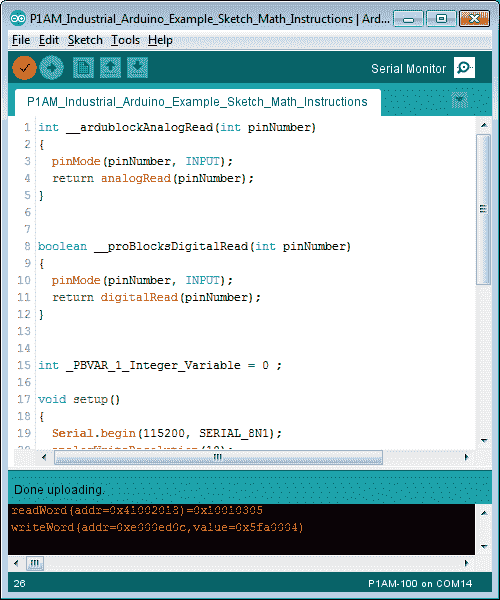Once the upload to the P1AM CPU is complete, we can select a serial monitor.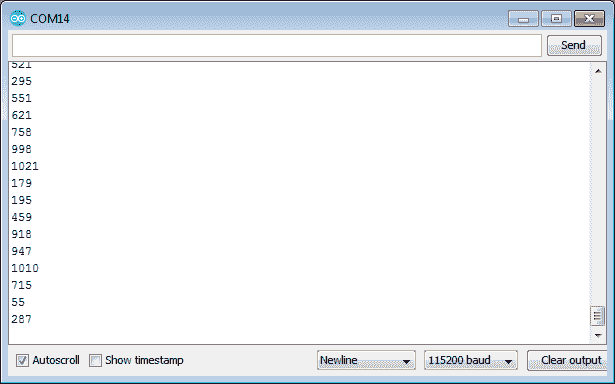Turn on the CPU switch and we will see our random number for the PWM output D2.

Watch the video below to see the operation of our sample math program with the productivity open industrial arduino controller.

Product Hardware
Productivity Open (Automation Direct)
P1AM-100 Specifications
Productivity Open User Manual
Configure a Productivity Open Arduino-based Controller
Open Source Controllers (Arduino-Compatible)
Productivity Open Documentation (Facts Engineering)
P1AM Design Files
Software
Arduino IDE (Integrated Development Environment)
P1AM-100 library (Easy Interface for controlling P1000 Modules)
Productivity Blocks (Development Timesaver)
Productivity Blocks Documentation (Wiki)
Community
Automation Direct Forum – Open Source Devices

Next time we will look at the time instructions using Productivity Blocks on the P1AM-100 Arduino Industrial Controller.

Watch on YouTube: Productivity Open P1AM Industrial Arduino Math Instructions